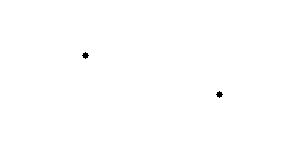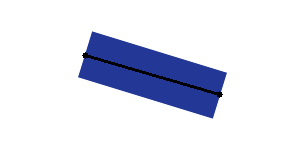# Create plane from 2 Vectors of a symmetry line segment

I have a text file that defines pairs of points (Vector3) that are related to one of the symmetry line segements that exists on a plane. The width and height of these planes are fixed and so, I want to create a plane given these points. This way, given a point A and a point B, I should be able to create the plane and rotate it in order that in the end, the points A and B form a symmetry line segment of the plane. How can i do that?

EDIT

This is what I have:This is what I want:But I just want the plane, not the line. The line is only to illustrate what the points mean to the plane, nothing more.

In solving this question there are three things that must be done, positioning the plane, scaling the plane, and rotating the plane. Each issue depends on the underlying plane and the your setup. I’m going to assume you are using the built-in Quad which is 1x1 units when scaled to (1,1,1). I’m going to assume that the camera is looking towards positive ‘z’. And I’m arbitrarily going to assume that you will align the top and the bottom of the of the plane with the points. Because you are aligning the top and the bottom, you scale the width (x) to anything you want.

You want to position the plane at the mid-point. Assuming your points are Vector3s name P1 and P2:

``````transform.position = P1 + (P2 - P1) / 2.0;
``````

Scaling:

``````transform.localScale.y = (P2 - P1).magnitude;
``````

Rotation:

``````transform.up = (P2 - P1);
``````

or:

``````transform.rotation = Quaternion.FromToRotation(transform.up, (P2 - P1));
``````

Putting it all together might look like:

``````var P21 = P2 - P1;
transform.position = P1 + P21 / 2.0;
transform.localScale = P21.magnitude;
transform.up = P21;
``````

Note if you are working in C#, you will have to save localScale to a temporary variable, change the ‘y’ parameter of the temporary variable, and assign it back.

This all assumes the setup is as I’ve described. Other setups may require a bit more code. For example, if the camera is point at the points from an arbitrary direction, you will likely need to some sort of billboarding.